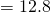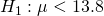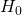# 2013 A-level H2 Mathematics (9740) Paper 2 Question 9 Suggested SolutionsAll solutions here are SUGGESTED. Mr. Teng will hold no liability for any errors. Comments are entirely personal opinions.

9
(i)
From the graphing calculator,

unbiased estimate of population meanunbiased estimate of population variance(ii)
We assume that 2.31 is a good estimate of the unknown population variance.
We assume the distance travelled per litre of fuel by a car is independent of another car.Under, perform a left-tailed T-test.

Test statistic,Using the graphing calculator, p-value, thus we do not reject.

There is insufficient evidence at 5% significance level to say that the distance travelled per litre of fuel is too high.

Not readable? Change text.